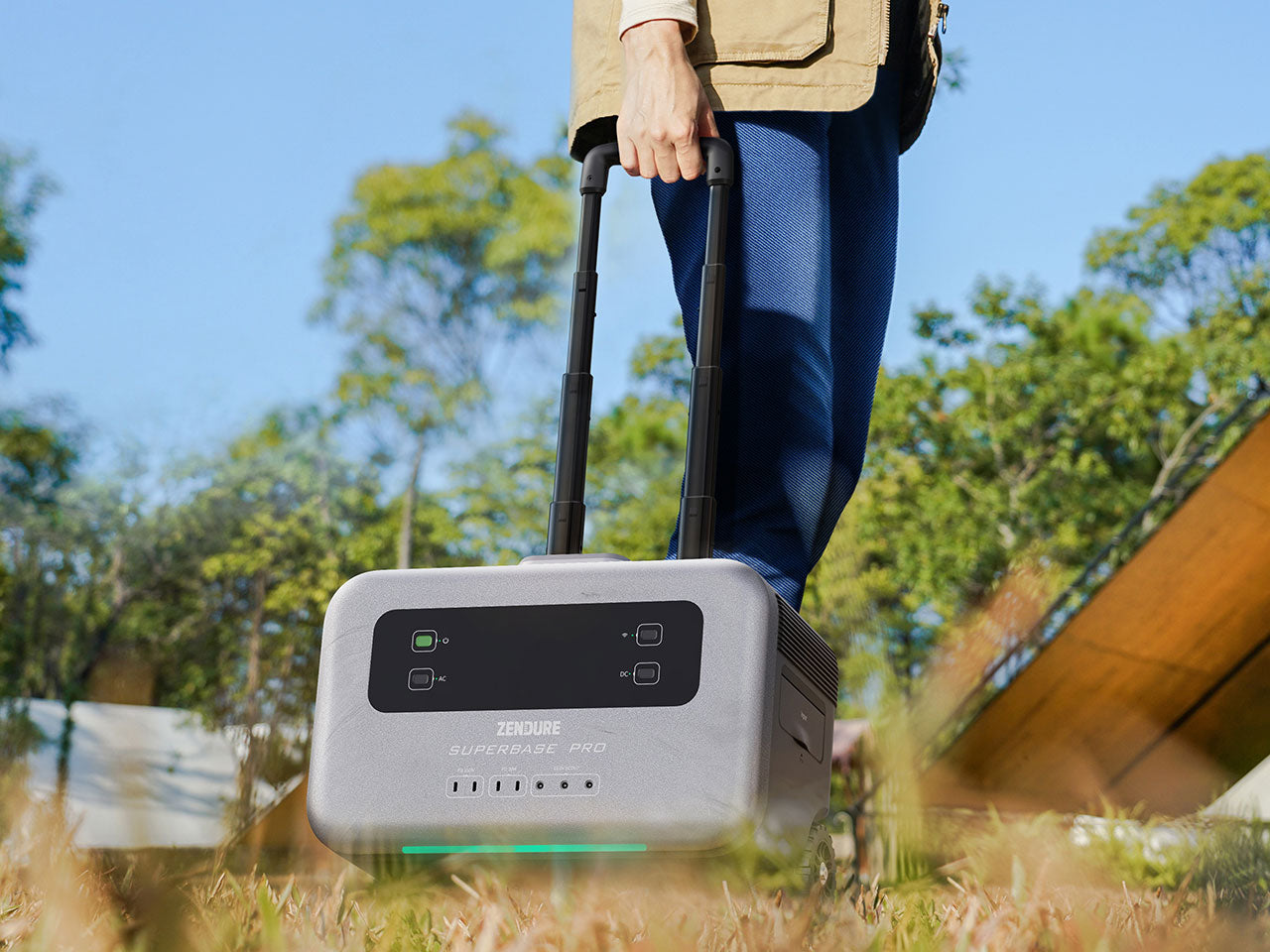Choose another country or region to see content specific to your location and shop online.

\$500 Limited-Time Offer on SuperBase V with code "ZDCPSBV500"!

﻿The term watt-hour, or Wh, is something you will see a lot when dealing with solar power battery packs and generators. It is an important unit of measurement that helps you understand the output and reliability of a product. So, what is a watt-hour, how do you calculate watt-hours, and why is it so important?

## What Is A Watt-Hour?

Before we figure out how to calculate watt-hours, it helps to get a better idea of the terminology. A watt-hour equates to one watt of power that is expended over a single hour. You will see this term a lot when dealing with energy outputs for electrical systems like generators and power packs. Rather than use the full name, companies will express this as a Wh unit.## Units Related To Watt-Hour

### 1) Ampere

This is the amount of energy flowing through the device per second. The higher the amps, the higher the current. This is a variable figure, whereas the voltage is fixed.

### 2) Volt Or Voltage

This is the amount of energy given to an electronic circuit or device from the battery. The batteries have a specific voltage, so you should always know what you are working with. This could be as low as 12V or maybe as high as 6V Either way, you need to be sure your device is compatible.

### 3) Watts

Watts relate to the power level of a device at a specific moment, whereas the watt-hours cover power consumption over a longer period. Both are helpful figures to know when dealing with solar power generators, but you still need to know the difference.

## How To Calculate Watt-Hours?

This all leads to an equation where energy is equal to power multiplied by time. In this case, you have your power in watts and time in hours, so your energy in watt-hours is watts multiplied by hours. For example, if you have a 150w solar panel running for 4 hours, that's 150 x 4, which is 600Wh.

To do this you need to be sure of both figures, and this can be tricky depending on the device and time period. For example, you may know the volts and amperes of a battery, but not the watts. If so, all you need to do is multiply the volts and amperes together. Alternatively, you can multiply the amp hours on a battery with the voltage to get the watt-hours. More on that later.## What Do Watt-Hours Have To Do With A Portable Power Station?

The reason it is so important to understand watt-hours is this is an essential detail when choosing portable power stations. The amount of energy you can store in a portable battery pack is expressed in Wh or kWh. The higher the number, the more watts it can deliver over a shorter period.

This extra power can make all the difference when running heavy-duty appliances during a blackout, such as fridges or medical equipment. It also means you can run more devices simultaneously without any issues. This is great when trying to maintain a sense of normalcy in an emergency, but also helps for recreational activities like camping.

## How To Calculate Watt-Hours Of A Battery?

Finally, we need to look at how to calculate the watt-hours of your batteries in case of these emergency situations. A lithium-ion battery tends to have a mAh rating as standard. It may also state the Wh but don't expect that.

First, you need to convert this mAh down to an Ah reading. This is simple enough as mAh means milliamps, and you can divide the number by 1000. A 4,400 mAh battery then converts to 4.4Ah. Then you can revert back to the formula above, with the amp hours multiplied by the voltage to get the watt-hours.

## How Many Watt-Hours In A 100 Ah Battery?

If you have a 100-ah battery, you need to multiply this by the voltage to get your watt-hours. A 100Ah 12V battery would be 1200Wh. A more powerful voltage, such as a 24V battery, would mean 2400Wh.

This all sounds like a lot to take in, and a lot of tricky calculations, but don't worry. If you can deal with the basic conversions and pay attention to the watt-hour rating on your power pack, you will be fine.